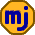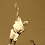## Thursday, December 3, 2009

### The Impact of MERs on Mutual Fund Returns

In a recent post, Canadian Capitalist showed that the effect of the HST on investor returns in mutual funds is small compared to the drag caused by fund MERs. I thought that while this conclusion is correct, his calculation of the MER impact was a little off. It turns out that we were both (slightly) wrong.

In the example, Investor A puts \$100 to work in the equity market for 25 years at an average annual return of 8%, giving a final portfolio value of \$685. Investor B makes a similar \$100 investment in a mutual fund with a 2.5% MER. Reasoning that Investor B’s return dropped to an average of 5.5% per year, his final portfolio value works out to \$381.

With the HST, the MER drag rises to 2.7% leaving 5.3% average return each year, and the final portfolio value is \$363. So, compared to Investor A’s \$685, the MER costs Investor B \$304, and the HST costs him another \$18. It’s clear that while the HST isn’t helping, the real problem is the high MER.

At first I thought that these calculations were a little off because the MER would knock 2.5% off the year-ending portfolio size, not the year-starting portfolio size. With this reasoning, Investor B’s return would be calculated as follows:

(1 + 0.08)*(1 – 0.025) = 1.053

This gives a 5.3% average return each year. Adding in the effect of the HST, we replace 0.025 with 0.027 and the average annual return drops to 5.084%.

It turns out that the truth is between Canadian Capitalist’s method and mine because of the way that the MER is reported. Mutual funds take the total costs of running the fund during the year and divide by the average assets under management during the year. The result is the MER percentage.

As it turns out, to calculate the effect of the MER on investor returns, you’ll need the e-to-the-power-of-x button on a scientific calculator or the EXP() function on a spreadsheet. For a detailed explanation of where the formula I’m about to use comes from, see the math section at the end of this post.

Here is how you can find the average yearly return after the MER is deducted:

(1 + 0.08)*(e^(–0.025)) = 1.05333

If we assume that the HST is deducted daily along with the MER, this changes to

(1 + 0.08)*(e^(–0.027)) = 1.05123

So, the average annual return is 5.333% without the HST and 5.123% with the HST. The portfolio ending value for Investor B is \$367 without the HST and \$349 with the HST. Compared to Investor A’s \$685, the MER costs \$318, and the HST costs an extra \$18.

The conclusion hasn’t changed: The HST is still a minor concern compared to MERs. But, I’ve learned something new about MERs as a result of seeking precision in these calculations. Canadian Capitalist is working on a spreadsheet that takes into account more details of how mutual funds are actually run to see what effect MERs have on returns.

The Math

Given the daily values of an index (including dividends) for a year, we’re looking for the returns generated by a mutual fund 100% invested in this index. We’ll assume that there are no net fund inflows or outflows each day of the year.

Some definitions:

m – MER (expressed as a fraction)
n – number of days in a trading year
A – average assets under management
C – total fund costs removed from the fund during the year
R – index return for the year
F – fund return for the year
v0, v1, ..., vn – index values over one year (v0 is starting value on the first day, v1 is the closing value on first day and the opening value on the second day, etc.)
f0, f1, ..., fn – corresponding fund values over one year

The average assets under management (based on each day’s closing value) are

A = (f1 + f2 + ... + fn)/n.

The one day share of the MER is m/n. Assuming the MER is taken out based on each day’s closing values, the MER for the first day is (m/n)f1. Continuing this way, the total costs for the year are

C = (m/n)*f1 + (m/n)*f2 + ... + (m/n)*fn = mA

Sanity check: MER = C/A = mA/A = m

On the first day the index rose by a factor of v1/v0. The same thing will happen to the fund on the first day before costs. On the first day, before costs are deducted, the fund will grow to

f0*(v1/v0)

The costs will be m/n times this quantity. After costs, the fund will hold

f1 = f0*(v1/v0)*(1–m/n)

On the second day, the fund grows by a factor of v2/v1 and costs shrink it by a factor of 1–m/n:

f2 = f0*(v2/v0)*(1–m/n)^2

Continuing this way we get

fn = f0*(vn/v0)*(1–m/n)^n

The index return for the year is calculated from the index starting and ending values for the year:

vn/v0 = 1+R

Similarly, for the fund:

fn/f0 = 1+F

Substituting these into the previous equation gives

1+F = (1+R)*(1–m/n)^n

That last factor looks intimidating, but as n becomes large, it approaches e^(-m). In this case, the number of days in a year is large enough that we can use the close approximation

1+F = (1+R)*(e^(–m))

For n=250 and m=0.025, the estimate differs from the real value by only 0.0000012. In Canadian Capitalist’s example, the difference between using the estimate and using the real equation over the 25 years is just over one cent.

If we assume that HST is paid each day, then we can just replace m by m*1.08 in the previous equation. If the HST payments are delayed, then the analysis changes a little, but the final result won't be much different.

1.WO! Slow Down there Math Man!

So the HST is charged against the MER itself, so if there was NO MER I wouldn't need to pay the HST? Hmmm.....

2.Big Cajun Man: I stick it at the end so that those who aren't interested can skip it. You did get the message from the first half loud and clear: HST wouldn't be a problem if the MER wasn't so high.

CC: If I understand you correctly, the return seen by an ETF owner who reinvests dividends immediately would be slightly higher than the reported ETF returns for the year. That's interesting.

It's true that MER costs are removed from a fund continuously throughout the year and thus the missed income represents an opportunity cost. That's why you can't take the index return and just subtract the MER to get the fund's return.

1.Thanks for the mention Michael. I did some more research on this topic and here's what I found out. Mutual funds and ETFs report total return as (ending value - starting value + income) / starting value. These dividends may be paid every quarter throughout the year but they are not assumed to be reinvested immediately.

Since costs are deducted from the cash balance, equity funds distributions are reduced by the extent of their expenses such as management fees, operating expenses and trading costs.

However, this is a real cost to investors. Personally, I reinvest dividends within a month or two of payment and since stock market returns are generally positive, on average, the high fund fees impose an opportunity cost on investors.

3.Great analysis. Reminded me of a quote from The Big Lebowski "He's a good man, and thorough."

Instead of doing the math, sometimes I compare the e-series and I-series index funds from TD. For example, TDB216 (Canadian Index, MER=0.84%) and TDB900 (Canadian Index, MER=0.31%). I don't know for certain that the two funds are identical, but whenever I've compared their holdings they are exactly the same. Over longer time-frames the e-series fund always leads the more expensive I-series fund. Here is a chart in Google Finance.

Equations are a quick way to kill the topic around some members of my family. So, do you think this visual approach is a valid way to demonstrate the impact of MERs to those who are less math inclined?

4.Ace. Thanks. Anything that gets the point across to the maximum number of people sounds like a good idea to me. I'm sure that my more mathematical posts are less interesting to a broader audience. It would be nice to show the effect of larger MERs, but your comparison, especially over a long period of time, works nicely.